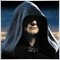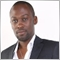# How to get Profit in Pips?60
10265293
Hi All,

how to get profit in pips?

OrderProfit() just for amount deposit, how do we get the profit in Pips?

i tried to use MathRound(OrderProfit()-OrderSwap())/(OrderLots()*MarketInfo(OrderSymbol(),MODE_TICKVALUE)), but not so accurate!!!!!57

dude, the profit in pips is not the difference between the OrderOpenPrice and OrderClosePrice (that's for closed orders; or the current price for opened ones)?151

```int start()
{
int D_Factor = 1;
double
Profit_Pips;
//----
if(Digits == 3||Digits == 5)D_Factor = 10;
for(int i=0;i<OrdersTotal();i++){
if(OrderType() == OP_BUY) Profit_Pips = (MarketInfo(OrderSymbol(), MODE_BID) - OrderOpenPrice())/Point/D_Factor;
if(OrderType() == OP_SELL) Profit_Pips = (OrderOpenPrice() - MarketInfo(OrderSymbol(),MODE_ASK))/Point/D_Factor;
Print("Order No : " + OrderTicket() + " Profit = " + DoubleToStr(Profit_Pips,1));
}
else{
break;
}
}
//----
return(0);
}```
For an open order, use the difference between current price(Bid/Ask) and the OrderOpenPrice.1091

kennyhubbard:
For an open order, use the difference between current price(Bid/Ask) and the OrderOpenPrice.

Kenny, I am assuming this is a Script not an EA. (If it's an EA it will run for ever and fill the logs.)

I notice that you used Point rather than

MarketInfo( OrderSymbol(), MODE_POINT );

which is a bug.

If there are any orders which are not OP_BUY or OP_SELL then you either print an uninitialised variable or the previous value, both of which are unpleasant.10

`int incomePips = (int) ((OrderProfit() - OrderSwap() - OrderCommission()) / OrderLots());`